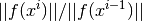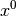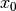sfepy.solvers.nls module¶

Nonlinear solvers.

class sfepy.solvers.nls.Newton(conf, **kwargs)[source]

Solves a nonlinear systemusing the Newton method.

The solver uses a backtracking line-search on divergence.

Kind: ‘nls.newton’

For common configuration parameters, see Solver.

Specific configuration parameters:

Parameters: i_max : int (default: 1) The maximum number of iterations. eps_a : float (default: 1e-10) The absolute tolerance for the residual, i.e.. eps_r : float (default: 1.0) The relative tolerance for the residual, i.e.. eps_mode : ‘and’ or ‘or’ (default: ‘and’) The logical operator to use for combining the absolute and relative tolerances. macheps : float (default: 2.2204460492503131e-16) The float considered to be machine “zero”. lin_red : float (default: 1.0) The linear system solution error should be smaller than (eps_a * lin_red), otherwise a warning is printed. lin_precision : float or None If not None, the linear system solution tolerances are set in each nonlinear iteration relative to the current residual norm by the lin_precision factor. Ignored for direct linear solvers. ls_on : float (default: 0.99999) Start the backtracking line-search by reducing the step, ifis larger than ls_on. ls_red : 0.0 < float < 1.0 (default: 0.1) The step reduction factor in case of correct residual assembling. ls_red_warp : 0.0 < float < 1.0 (default: 0.001) The step reduction factor in case of failed residual assembling (e.g. the “warp violation” error caused by a negative volume element resulting from too large deformations). ls_min : 0.0 < float < 1.0 (default: 1e-05) The minimum step reduction factor. give_up_warp : bool (default: False) If True, abort on the “warp violation” error. check : 0, 1 or 2 (default: 0) If >= 1, check the tangent matrix using finite differences. If 2, plot the resulting sparsity patterns. delta : float (default: 1e-06) If check >= 1, the finite difference matrix is taken as. log : dict or None If not None, log the convergence according to the configuration in the following form: {'text' : 'log.txt', 'plot' : 'log.pdf'}. Each of the dict items can be None. is_linear : bool (default: False) If True, the problem is considered to be linear.
__call__(vec_x0, conf=None, fun=None, fun_grad=None, lin_solver=None, iter_hook=None, status=None)[source]

Nonlinear system solver call.

Solves a nonlinear systemusing the Newton method with backtracking line-search, starting with an initial guess.

Parameters: vec_x0 : array The initial guess vector. conf : Struct instance, optional The solver configuration parameters, fun : function, optional The functionwhose zero is sought - the residual. fun_grad : function, optional The gradient of- the tangent matrix. lin_solver : LinearSolver instance, optional The linear solver for each nonlinear iteration. iter_hook : function, optional User-supplied function to call before each iteration. status : dict-like, optional The user-supplied object to hold convergence statistics.

Notes

• The optional parameters except iter_hook and status need to be given either here or upon Newton construction.
• Setting conf.is_linear == True means a pre-assembled and possibly pre-solved matrix. This is mostly useful for linear time-dependent problems.
__init__(conf, **kwargs)[source]
__metaclass__

alias of SolverMeta

__module__ = ‘sfepy.solvers.nls’
name = ‘nls.newton’
class sfepy.solvers.nls.PETScNonlinearSolver(conf, pmtx=None, prhs=None, comm=None, **kwargs)[source]

Interface to PETSc SNES (Scalable Nonlinear Equations Solvers).

The solver supports parallel use with a given MPI communicator (see comm argument of PETScNonlinearSolver.__init__()). Returns a (global) PETSc solution vector instead of a (local) numpy array, when given a PETSc initial guess vector.

For parallel use, the fun and fun_grad callbacks should be provided by PETScParallelEvaluator.

Kind: ‘nls.petsc’

For common configuration parameters, see Solver.

Specific configuration parameters:

Parameters: method : str (default: ‘newtonls’) The SNES type. i_max : int (default: 10) The maximum number of iterations. if_max : int (default: 100) The maximum number of function evaluations. eps_a : float (default: 1e-10) The absolute tolerance for the residual, i.e.. eps_r : float (default: 1.0) The relative tolerance for the residual, i.e.. eps_s : float (default: 0.0) The convergence tolerance in terms of the norm of the change in the solution between steps, i.e. $||delta x|| < epsilon_s ||x||$
__call__(vec_x0, conf=None, fun=None, fun_grad=None, lin_solver=None, iter_hook=None, status=None, pmtx=None, prhs=None, comm=None)[source]
__init__(conf, pmtx=None, prhs=None, comm=None, **kwargs)[source]
__metaclass__

alias of SolverMeta

__module__ = ‘sfepy.solvers.nls’
name = ‘nls.petsc’
class sfepy.solvers.nls.ScipyBroyden(conf, **kwargs)[source]

Interface to Broyden and Anderson solvers from scipy.optimize.

Kind: ‘nls.scipy_broyden_like’

For common configuration parameters, see Solver.

Specific configuration parameters:

Parameters: method : str (default: ‘anderson’) The name of the solver in scipy.optimize. i_max : int (default: 10) The maximum number of iterations. alpha : float (default: 0.9) See scipy.optimize. M : float (default: 5) See scipy.optimize. f_tol : float (default: 1e-06) See scipy.optimize. w0 : float (default: 0.1) See scipy.optimize.
__call__(vec_x0, conf=None, fun=None, fun_grad=None, lin_solver=None, iter_hook=None, status=None)[source]
__init__(conf, **kwargs)[source]
__metaclass__

alias of SolverMeta

__module__ = ‘sfepy.solvers.nls’
name = ‘nls.scipy_broyden_like’
set_method(conf)[source]
sfepy.solvers.nls.check_tangent_matrix(conf, vec_x0, fun, fun_grad)[source]

Verify the correctness of the tangent matrix as computed by fun_grad() by comparing it with its finite difference approximation evaluated by repeatedly calling fun() with vec_x0 items perturbed by a small delta.

sfepy.solvers.nls.conv_test(conf, it, err, err0)[source]

Nonlinear solver convergence test.

Parameters: conf : Struct instance The nonlinear solver configuration. it : int The current iteration. err : float The current iteration error. err0 : float The initial error. status : int The convergence status: -1 = no convergence (yet), 0 = solver converged - tolerances were met, 1 = max. number of iterations reached.